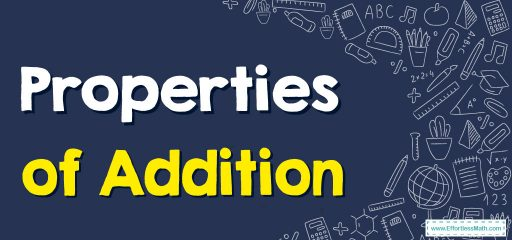This guide focuses on providing comprehensive information on the various Properties of Addition. The properties of addition are fundamental mathematical concepts that are used in various mathematical operations.## A step-by-step guide to solving Properties of Addition problems

Properties of addition refer to the rules and laws that govern the behavior of addition in mathematics. These properties help simplify arithmetic operations and make it easier to evaluate complex expressions. Some of the commonly recognized properties of addition include:

1. Commutative Property: This property states that changing the order of the numbers being added does not affect the result. For example, 3 + 4 = 4 + 3.
2. Associative Property: This property states that changing the grouping of the numbers being added does not affect the result. For example, (2 + 3) + 4 = 2 + (3 + 4).
3. Identity Property: This property states that adding zero to any number does not change the value of that number. For example, 4 + 0 = 4.
4. Inverse Property: This property states that every number has an opposite, and when the opposite is added to the original number, the result is zero. For example, 4 + (-4) = 0.

These properties of addition are essential to understand in order to simplify complex expressions and solve problems related to addition.

### Properties of Addition – Example 1

Which property of addition is shown in the equation below?
$$(k+w)+8=k+(w+8)$$
Solution:
Since the numbers are re-grouped, it is the associative property.

### Properties of Addition – Example 2

Which property of addition is shown in the equation below?
$$c+7+n=c+n+7$$
Solution:
Since the numbers are moved around, it is the commutative property.

### What people say about "Properties of Addition - Effortless Math: We Help Students Learn to LOVE Mathematics"?

No one replied yet.

X
30% OFF

Limited time only!

Save Over 30%

SAVE $5 It was$16.99 now it is \$11.99